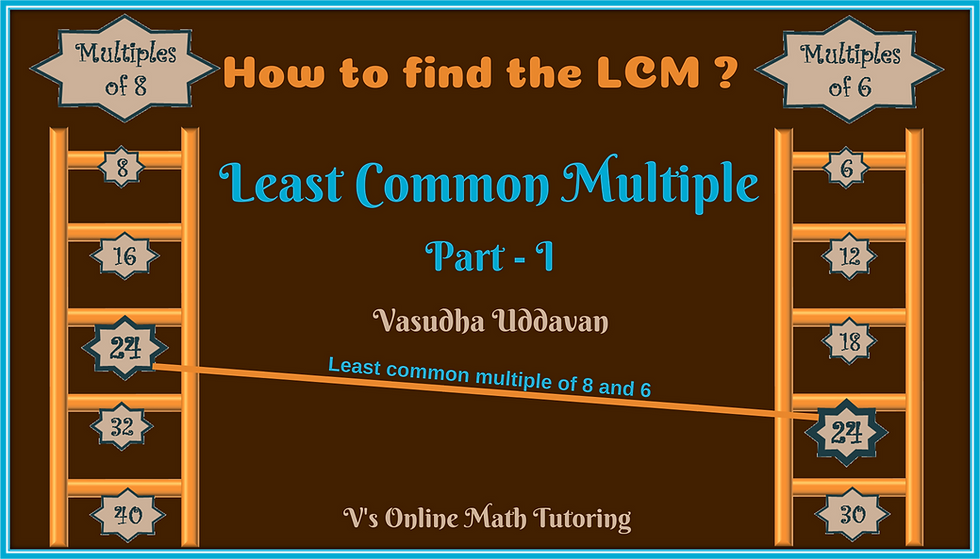top of pageSearch

# How to find the LCM (Least Common Multiple) and how I teach it - Part 2

Updated: Nov 29, 2018

As you saw from Part 1 of this post, finding LCM by just listing the multiples is very easy as long as the numbers are small. What happens if you have to find the LCM of larger numbers? Even LCM of numbers like 24 and 40. The listing method becomes tedious. So is there another way? Of course there is and we are going to look at the L-method of finding LCM today.Second Method: The L-method

Example 1 - Let's find the LCM of 6 and 8Least Common Multiple of 6 and 8

First we draw an L and write the 2 number - 6&8 for which we are trying to find the LCM

Then we find a common factor for 6 and 8 - which is 2 and we write that to the left of the L drawn.

Then we write how many times the common factor will go into 6 and 8 below them.

So we get 3 and 4. Since 3 and 4 do not have any common factors we stop here

The LCM of 6 and 8 is 2 * 3 * 4 = 24

Example 2 - Find the LCM of 13 and 15Least Common Multiple (LCM) of 13 and 15

Once again we draw an L and write the 2 number - 13 &15 for which we are trying to find the LCM

Then we find a common factor for 13 and 15.

Since they do not have any common factors, the LCM is 13 * 15 = 195

Did you see how easy that was? No listing at all. Easy peasy :)

Does it work for larger numbers? Sure.

Example 3: Find the LCM of 40 and 100.Least Common Multiple LCM of 40 and 100

Once again we draw an L and write the 2 number - 40 & 100 for which we are trying to find the LCM

Then we find a common factor for 40 and 100. 5 is a common factor.

Then we write how many times the common factor 5 will go into 40 and 100 below them.

5 goes into 40, 8 times and 5 goes into 100, 20 times. So we write 8 and 20 below 40 and 100.

Do 8 and 40 have any more common factors? Yes, 4 is a common factor.

Then we write how many times the common factor 4 will go into 8 and 20 below them.

So we get 2 and 5. And since 2 and 5 do not have any common factors, we stop here

Hence LCM of 40 and 100 = 5*4*2*5 = 200How to find the LCM of 6 & 8

If the L- method is easy, why did we look at the listing method? Because it helps in understanding exactly what LCM means. Once you've understood what LCM is then almost everyone uses the L-method for finding LCM.

Now try a problem thats popular in MOEMS (Math Olympiad for Elementary and Middle Schools) and other math competitions.

Problem: Find the smallest number that leaves a remainder of 5 when divided by 40 and 100.Problem that uses LCM

Related Posts:

How to find the LCM - Part 1How to find the LCM - Listing Method